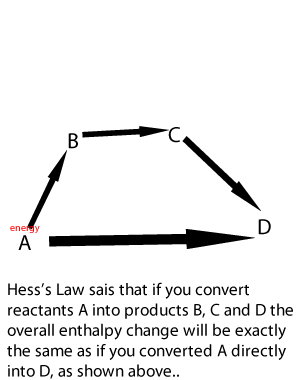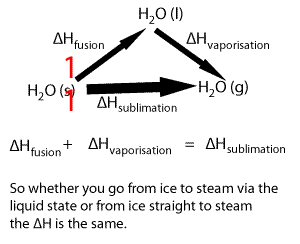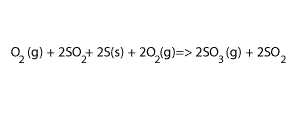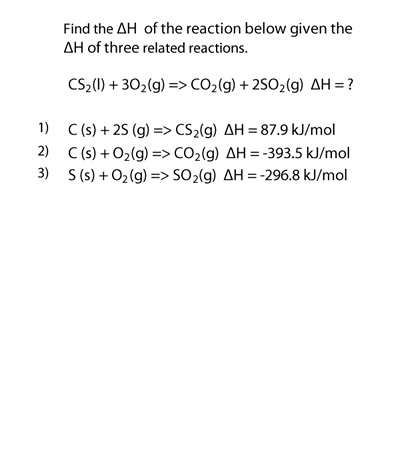Enthalpy calculationsWe can deduce the ΔH of an overall reaction given energy profiles or given the ΔH of related reactions. The ΔH of any process depends on the initial and final stages of that process. We can illustrate this simply by looking at the ΔH of the sublimation of water, that is water going from a solid straight to the gaseous state. Lets take the following equations 1) ---- H2O(s) => H2O(l) ΔH = 6.00 kJ/mol 2) ---- H2O(l) => H2O(g) ΔH = 44.01 kJ/mol Adding 1) and 2) we get H2O(s) + H2O(l) => H2O(l) + H2O(g) ΔH = 50.01 kJ/mol Now since H2O(l) appears on both sides of the equation we can cancel it out to get the equation below H2O(s) => H2O(g) ΔH = 50.01 kJ/molLets try some examples. But before we do remember: - if you reverse the reaction the sign of the ΔH must change, - if you add two reactions you add their ΔH, - if you multiply an equation you must also multiply the ΔH. Example 1 Find the ΔH of the reaction below CO 2(g) + H 2(g) => CO(g) + H 2O(g) given the two equations below. 1) ----- 2H 2(g) + O 2(g) => 2H 2O(g) ΔH = - 484 kJ/mol 2) ----- 2CO(g) + O 2(g) =>2CO 2(g) ΔH = - 566 kJ/mol Step 1 Reverse equation 2) 3) ----- 2CO 2(g) => 2CO(g) + O 2(g) ΔH = 566 kJ/mol Step 2 Add equations 1) and 3) and dont forget to also add their ΔH 4) ----- 2CO 2(g) + 2H 2(g) => 2CO(g) + 2H 2O(g) ΔH = (566 - 484) = 82 kJ/mol Step 3 Divide equation 4) by 2 and do the same to its ΔH CO 2(g) + H 2(g) => CO(g) + H 2O(g) ΔH = 82/2 = 41 kJ/mol Example 2 Calculate the ΔH of the reaction 2S(s) + 3O 2(g) =>2SO3(g) given 1) ----- S(s) + O 2(g) => SO2(g) ΔH = - 297 kJ/mol 2) ----- 2SO3(g) =>O 2(g) + 2SO2 (g) ΔH = 196 kJ/mol Step 1 Reverse equation 2) to eliminate the SO2 and don't forget to change the sign of the ΔH . 3) ----- O2(g) + 2SO2 => 2SO3(g) ΔH = - 196 kJ/mol Step 3 Multiply equation 1) by 2 and don't forget to multiply the ΔH 4) ----- 2S(s) + 2O 2(g) => 2SO2(g) ΔH = - 594 kJ/mol Step 4 Add equations 3) and 4) and cancel any substance that appears on both sides, as shown on the right. Also add their ΔH 3O2(g)+2S(s) + 2O 2(g) => 2SO3(g) ΔH = - 790 kJ/mol1) Calculate the ΔH of the direct synthesis of methanol according to the equation 2C(s) + O 2(g) + 4H2(g) => 2CH3OH (g) ΔH = ? given the ΔH of the following reactions. 1) ----- 2CH3OH (g) + 3O 2(g) => 4H2O(g) + 2CO2 (g) ΔH = - 1352 kJ/mol 2) ----- C(s) + O 2(g) => CO2(g) ΔH = - 393 kJ/mol 3) ----- 2 H2(g) + O 2(g) => 2H2O(g) ΔH = - 484 kJ/mol Solution 2) Calculate the ΔH of the following reaction 2CO(g) + O2(g) => 2CO2 (g) given 1) ----- C(s) + O 2(g) => CO2(g) ΔH = - 393 kJ/mol 2) ----- 2C(s) + O 2(g) => 2CO(g) ΔH = - 221 kJ/mol 3) Calculate the ΔH of the following reaction 2N2 (g) + 5O2(g) => 2N2O5 (g) given 1) ----- 2 H2(g) + O2 (g) => 2H2O(l) ΔH = - 571.6 kJ/mol 2) ----- N2O5 (g) + H2O(l) =>2HNO3(l) ΔH = - 76.6 kJ/mol 3) ----- N2 (g) + 3O2 (g) + H2 (g) =>2HNO3(l) ΔH = - 348.2 kJ/mol4) Consider the exercise on the left. Solution Step 1 Step 2 Step 3 Continue with an activity